## 求一个多表多字段查询sql语句

Photon 发布于 2013/02/21 09:39

2张表A和B，A表column1跟B表column1比较，如果有结果就把A表column2查出来，没结果就跟B表column2比较，如果还没结果那么A表column2查出来就是null，最终数据量跟单独查询B表一样，所以我觉得应该是以B表为主表，left join A表，试了好多写法都不行，结果会多一些数据，求帮忙，多谢～～

0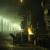0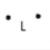#### 引用来自“amar”的答案

```select c.uniqueid, c.clid, c.dst, c.calldate, c.billsec, m.filepath, e.channel, e.account, case when c.channel = e.channel then e.account when c.dstchannel = e.channel then e.account else null end
from cdr c left join extension e on 1 = 1
and case when c.channel = e.channel then e.account when c.dstchannel = e.channel then e.account else null end
left join monitorfile m on m.uniqueid in (c.uniqueid, c.userfield)
order by c.calldate desc```
0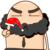```SELECT nvl( (select a.column1 from a where a.column1=b.column1),
nvl( (select a.column2 from a where a.column1=b.column2),
null
)
)
FROM b  where 1=1```
0#### 引用来自“throwable”的答案

```SELECT nvl( (select a.column1 from a where a.column1=b.column1),
nvl( (select a.column2 from a where a.column1=b.column2),
null
)
)
FROM b  where 1=1```

0#### 引用来自“amar”的答案

```select c.uniqueid, c.clid, c.dst, c.calldate, c.billsec, m.filepath, e.channel, e.account, case when c.channel = e.channel then e.account when c.dstchannel = e.channel then e.account else null end
from cdr c left join extension e on 1 = 1
and case when c.channel = e.channel then e.account when c.dstchannel = e.channel then e.account else null end
left join monitorfile m on m.uniqueid in (c.uniqueid, c.userfield)
order by c.calldate desc```

00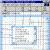0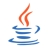select * from a left join b on a.c1 = b.c1 or a.c1 = b.c2 我觉得你可能当时思维混乱了下，题目逻辑很清楚以a为主了。